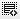## Rate of return2
How is rate of return for the investor calculated if we know the capital invested, interest earned and the duration of the investment?2

you can use the FV(future value)formula for this.

FV= PV(1+r)^n

PV= capital invested
FV= total int earned+ capital invested
r= rate of interest
n= duration

Click on thisicon to add code snippet.